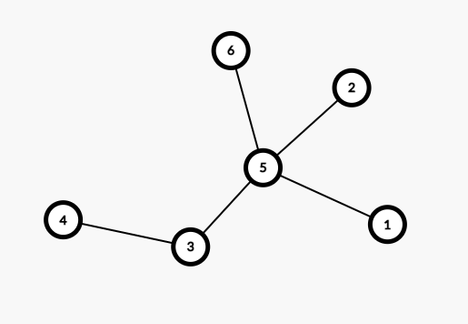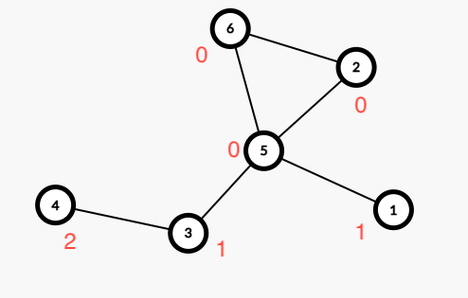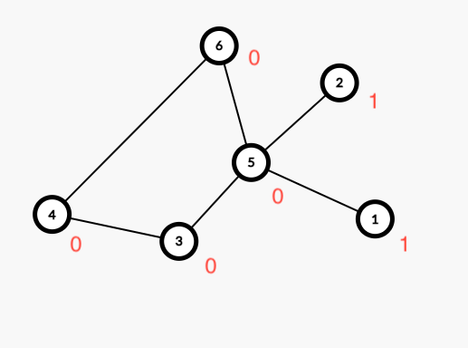# Holy Treeovis96Replay of Cefalo SUST Int...
Limits 1s, 512 MB

What is a tree?

A tree is a bidirectional connected graph with no cycle.

In a tree, we can create exactly one cycle if we connect any two nodes by an edge. Professor Ratman thinks that the cycle has holy powers. So the cost of such one cyclic graph is the total distance from all the nodes to any node of the cycle. Professor Ratman wants to determine the minimum cost given that you can connect any two nodes of the tree.

So you have to minimize $\sum(\text{distance from every node to the cycle})$

Suppose, we have the following tree:If we connect 2 and 6 and make a cycle, then the cost would be 1 + 1 + 2 = 4.But, if we connect 4 and 6, the cost will be, 1 + 1 = 2, which is the minimum cost in this case.Your job is to find the minimum cost of creating one cycle.

## Input

The first line will contain an integer $T ( 1 \leq T \leq 10)$, the number of test cases.

For each test case, the first line contains $n (1 \leq n \leq 10^5)$, the number of nodes in the tree.

Next $n-1$ lines will describe the tree. Each line will contain two space-separated integers $u$ and $v (1 \leq u, v \leq n)$ indicating that there is a bidirectional edge between $u$ and $v$.

It is guaranteed that the input is a valid tree and the sum of $n$ over all test cases will not exceed $3\times10^5$, that means $\sum\limits_{t=1}^{T}(n) \leq 3\times10^5$.

## Output

For each test case, print “Case X: Y” where “X” indicates the case number and “Y” indicates the minimum cost for the given case.

## Sample

InputOutput
1
6
1 5
5 2
5 6
3 5
3 4

Case 1: 2### Submit